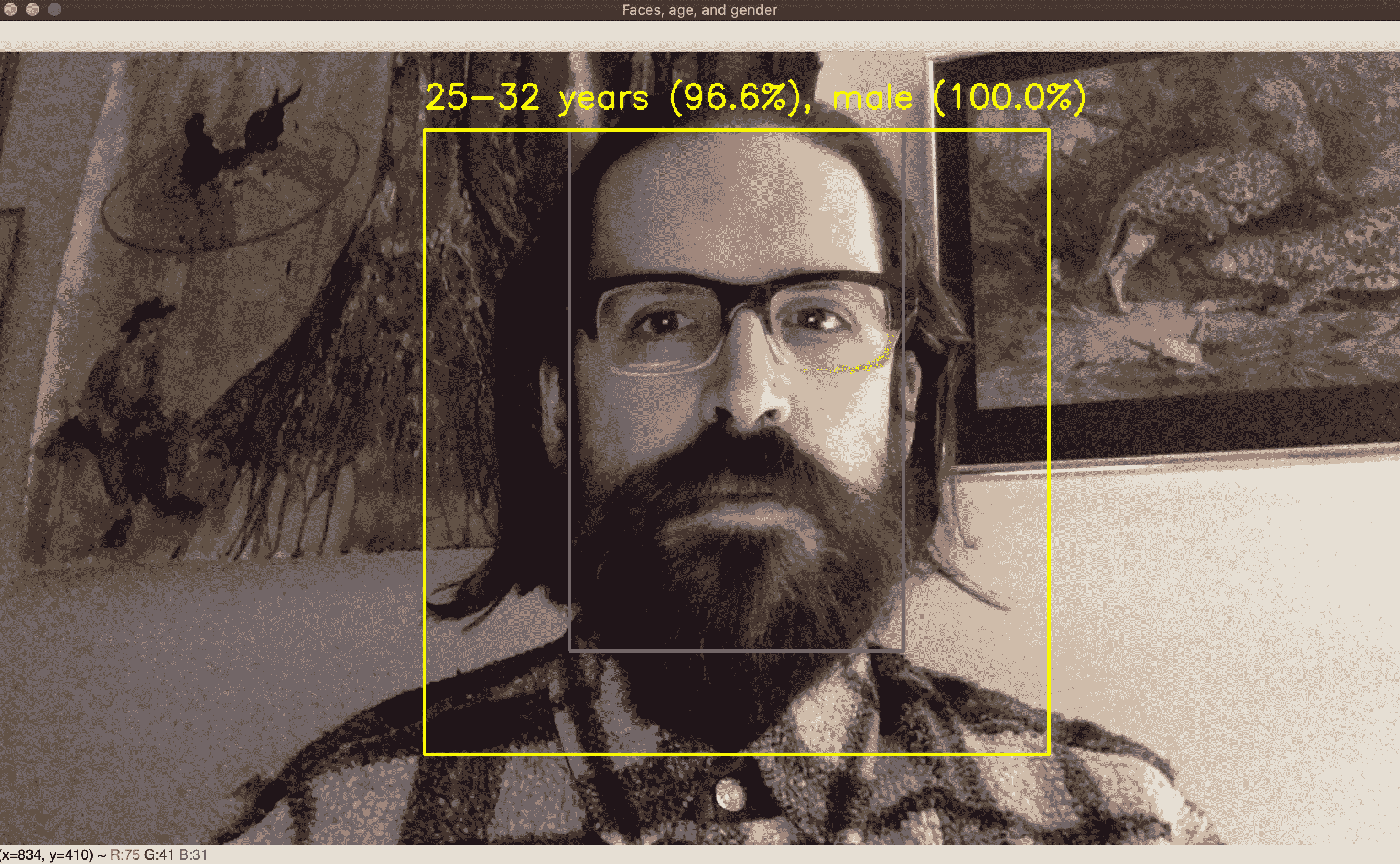# 十、使用 OpenCV 的神经网络简介

• 输入变量很多，它们之间可能具有复杂的非线性关系。
• 有许多输出变量，这些变量可能与输入变量具有复杂的非线性关系。 （通常，分类问题中的输出变量是类的置信度得分，因此，如果有很多类，那么会有很多输出变量。）
• 有许多隐藏的（未指定）变量可能与输入和输出变量具有复杂的非线性关系。 DNN 甚至旨在建模多个隐变量层，这些隐层主要彼此相关，而不是主要与输入或输出变量相关。

• 将人工神经网络理解为统计模型和有监督的机器学习工具。
• 了解 ANN 拓扑，或者将 ANN 组织到相互连接的神经元层中。 特别地，我们将考虑使 ANN 能够用作一种分类器的拓扑，称为多层感知器MLP）。
• 在 OpenCV 中训练和使用人工神经网络作为分类器。
• 生成检测和识别手写数字（0 到 9）的应用。 为此，我们将基于被广泛使用的称为 MNIST 的数据集训练 ANN，该数据集包含手写数字的样本。
• 在 OpenCV 中加载和使用经过预训练的 DNN。 我们将介绍 DNN 的对象分类，人脸检测和性别分类的示例。

# 了解人工神经网络

• P是复杂场景的简化。
• P是首先生成S或至少与S非常相似的一组观察结果的函数。

• 监督学习：在这种方法下，我们希望模型的训练过程产生一个函数，该函数将一组已知的输入变量映射到一组已知的输出变量。 我们知道，先验是预测问题的性质，我们将找到解决该问题的函数的过程委托给了 ANN。 要训​​练模型，我们必须提供输入样本以及正确的相应输出。 对于分类问题，输出变量可以是一个或多个类别的置信度得分。
• 无监督学习：在这种方法下，先验不知道输出变量的集合。 模型的训练过程必须产生一组输出变量，以及将输入变量映射到这些输出变量的函数。 对于分类问题，无监督学习可能导致发现先前未知的类别，例如医学数据中的先前未知的疾病。 无监督学习可以使用包括（但不限于）聚类的技术，我们在第 7 章，“构建自定义对象检测器”的 BoW 模型的上下文中对此进行了探讨。
• 强化学习：这种方法可以颠倒典型的预测问题。 在训练模型之前，我们已经有一个系统，当我们为一组已知的输入变量输入值时，该系统会为一组已知的输出变量产生值。 我们知道，先验是一种基于输出的优劣（合意性）或缺乏而对输出序列进行评分的方法。 但是，我们可能不知道将输入映射到输出的实际函数，或者，即使我们知道它，也是如此复杂，以至于无法为最佳输入求解。 因此，我们希望模型的训练过程能够产生一个函数，该函数根据最后的输出来预测序列中的下一个最优输入。 在训练过程中，模型从分数中学习，该分数最终是由其动作（所选输入）产生的。 从本质上讲，该模型必须学会在特定的奖惩系统中成为优秀的决策者。

# 了解神经元和感知器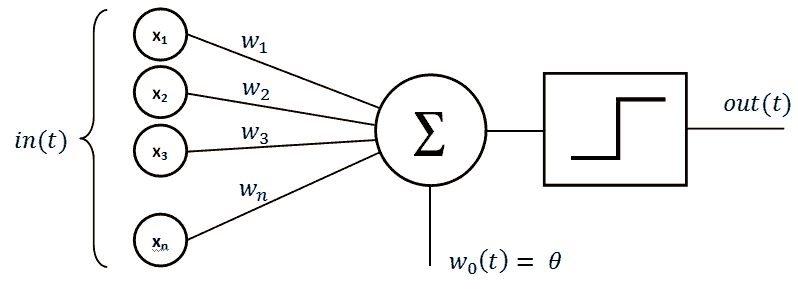# 了解神经网络的各层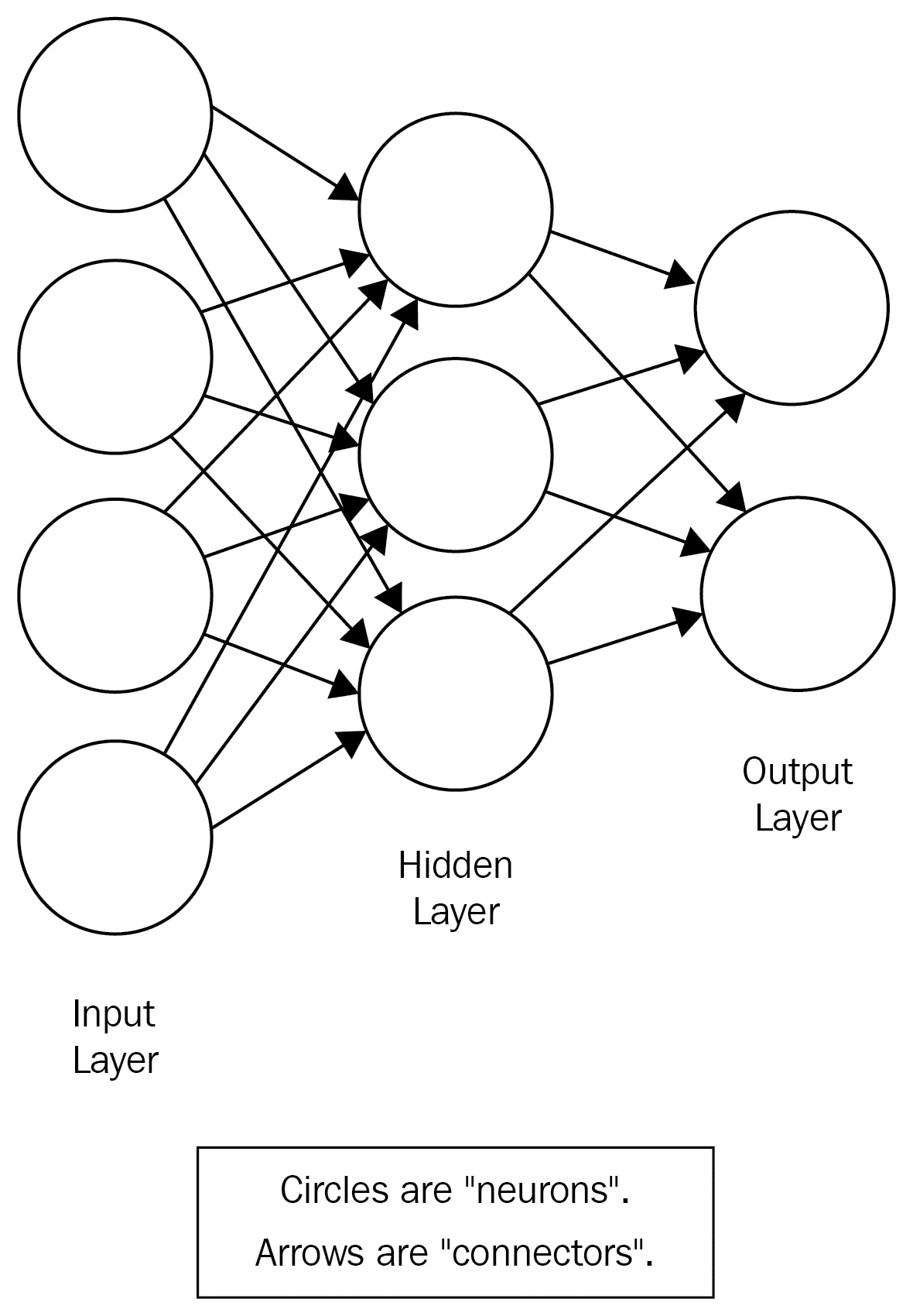# 选择隐藏层的大小

• 如果输入层很大，则隐藏神经元的数量应在输入层的大小和输出层的大小之间，并且通常应更接近输出层的大小。
• 另一方面，如果输入和输出层都较小，则隐藏层应为最大层。
• 如果输入层较小，但输出层较大，则隐藏层应更接近输入层的大小。

# 在 OpenCV 中训练基本的 ANN

OpenCV 提供了cv2.ml_ANN_MLP类，该类将 ANN 实现为多层感知器MLP）。 这正是我们之前在“了解神经元和感知器”部分中描述的模型。

1. 首先，我们照常导入 OpenCV 和 NumPy：
import cv2
import numpy as np

1. 现在，我们创建一个未经训练的人工神经网络：
ann = cv2.ml.ANN_MLP_create()

1. 创建 ANN 后，我们需要配置其层数和节点数：
ann.setLayerSizes(np.array([9, 15, 9], np.uint8))


1. 我们还可以配置激活函数，训练方法和训练终止标准，如下所示：
ann.setActivationFunction(cv2.ml.ANN_MLP_SIGMOID_SYM, 0.6, 1.0)
ann.setTrainMethod(cv2.ml.ANN_MLP_BACKPROP, 0.1, 0.1)
ann.setTermCriteria(
(cv2.TERM_CRITERIA_MAX_ITER | cv2.TERM_CRITERIA_EPS, 100, 1.0))


1. 让我们训练 ANN。 我们需要指定训练输入（或 OpenCV 术语中的samples），相应的正确输出（或responses），以及数据的格式（或layout）是每个样本一行还是每个样本一行。 这是一个如何使用单个样本训练模型的示例：
training_samples = np.array(
[[1.2, 1.3, 1.9, 2.2, 2.3, 2.9, 3.0, 3.2, 3.3]], np.float32)
layout = cv2.ml.ROW_SAMPLE
training_responses = np.array(
[[0.0, 0.0, 0.0, 0.0, 0.0, 1.0, 0.0, 0.0, 0.0]], np.float32)
data = cv2.ml.TrainData_create(
training_samples, layout, training_responses)
ann.train(data)


1. 为了完成对 ANN API 的最小介绍，让我们制作另一个示例，对其进行分类并打印结果：
test_samples = np.array(
[[1.4, 1.5, 1.2, 2.0, 2.5, 2.8, 3.0, 3.1, 3.8]], np.float32)
prediction = ann.predict(test_samples)
print(prediction)


(5.0, array([[-0.08763029, -0.01616517, 0.13196233, 0.0402631 , 0.05711843,
1.1642447 , 0.18130444, 0.1857026 , -0.07486832]],
dtype=float32))


# 在多个周期中训练 ANN 分类器

• 我们将增加隐藏层中神经元的数量。
• 我们将使用更大的训练数据集。 为方便起见，我们将随机生成此数据集。
• 我们将在多个周期训练 ANN，这意味着我们将使用相同的数据集每次对其进行多次训练和重新训练。

1. 首先，我们照例导入 OpenCV 和 NumPy。 然后，从 Python 标准库中，导入randint函数以生成伪随机整数，并导入uniform函数以生成伪随机浮点数：
import cv2
import numpy as np
from random import randint, uniform

1. 接下来，我们创建并配置 ANN。 这次，我们使用三个神经元输入层，一个 50 神经元隐藏层和一个四个神经元输出层，如以下代码中以粗体突出显示：
animals_net = cv2.ml.ANN_MLP_create()
animals_net.setLayerSizes(np.array([3, 50, 4]))
animals_net.setActivationFunction(cv2.ml.ANN_MLP_SIGMOID_SYM, 0.6, 1.0)
animals_net.setTrainMethod(cv2.ml.ANN_MLP_BACKPROP, 0.1, 0.1)
animals_net.setTermCriteria(
(cv2.TERM_CRITERIA_MAX_ITER | cv2.TERM_CRITERIA_EPS, 100, 1.0))

1. 现在，我们需要一些数据。 我们对准确地代表动物并不感兴趣。 我们只需要一堆记录作为训练数据即可。 因此，我们定义四个函数以生成不同类别的随机样本，另外定义四个函数以生成正确的分类结果以进行训练：
"""Input arrays
weight, length, teeth
"""

"""Output arrays
dog, condor, dolphin, dragon
"""

def dog_sample():
return [uniform(10.0, 20.0), uniform(1.0, 1.5),
randint(38, 42)]

def dog_class():
return [1, 0, 0, 0]

def condor_sample():
return [uniform(3.0, 10.0), randint(3.0, 5.0), 0]

def condor_class():
return [0, 1, 0, 0]

def dolphin_sample():
return [uniform(30.0, 190.0), uniform(5.0, 15.0),
randint(80, 100)]

def dolphin_class():
return [0, 0, 1, 0]

def dragon_sample():
return [uniform(1200.0, 1800.0), uniform(30.0, 40.0),
randint(160, 180)]

def dragon_class():
return [0, 0, 0, 1]

1. 我们还定义了以下辅助函数，以便将样本和分类转换为一对 NumPy 数组：
def record(sample, classification):
return (np.array([sample], np.float32),
np.array([classification], np.float32))

1. 让我们继续创建假动物数据。 我们将为每个类创建 20,000 个样本：
RECORDS = 20000
records = []
for x in range(0, RECORDS):
records.append(record(dog_sample(), dog_class()))
records.append(record(condor_sample(), condor_class()))
records.append(record(dolphin_sample(), dolphin_class()))
records.append(record(dragon_sample(), dragon_class()))

1. 现在，让我们训练 ANN。 正如我们在本节开头所讨论的，我们将使用多个训练周期。 每个周期都是循环的迭代，如以下代码所示：
EPOCHS = 10
for e in range(0, EPOCHS):
print("epoch: %d" % e)
for t, c in records:
data = cv2.ml.TrainData_create(t, cv2.ml.ROW_SAMPLE, c)
if animals_net.isTrained():
animals_net.train(data, cv2.ml.ANN_MLP_UPDATE_WEIGHTS | cv2.ml.ANN_MLP_NO_INPUT_SCALE | cv2.ml.ANN_MLP_NO_OUTPUT_SCALE)
else:
animals_net.train(data, cv2.ml.ANN_MLP_NO_INPUT_SCALE | cv2.ml.ANN_MLP_NO_OUTPUT_SCALE)


1. 训练完我们的人工神经网络后，我们应该进行测试。 对于每个类别，让我们生成 100 个新的随机样本，使用 ANN 对其进行分类，并跟踪正确分类的数量：
TESTS = 100

dog_results = 0
for x in range(0, TESTS):
clas = int(animals_net.predict(
np.array([dog_sample()], np.float32)))
print("class: %d" % clas)
if clas == 0:
dog_results += 1

condor_results = 0
for x in range(0, TESTS):
clas = int(animals_net.predict(
np.array([condor_sample()], np.float32)))
print("class: %d" % clas)
if clas == 1:
condor_results += 1

dolphin_results = 0
for x in range(0, TESTS):
clas = int(animals_net.predict(
np.array([dolphin_sample()], np.float32)))
print("class: %d" % clas)
if clas == 2:
dolphin_results += 1

dragon_results = 0
for x in range(0, TESTS):
clas = int(animals_net.predict(
np.array([dragon_sample()], np.float32)))
print("class: %d" % clas)
if clas == 3:
dragon_results += 1

1. 最后，让我们打印准确率统计信息：
print("dog accuracy: %.2f%%" % (100.0 * dog_results / TESTS))
print("condor accuracy: %.2f%%" % (100.0 * condor_results / TESTS))
print("dolphin accuracy: %.2f%%" % \
(100.0 * dolphin_results / TESTS))
print("dragon accuracy: %.2f%%" % (100.0 * dragon_results / TESTS))


dog accuracy: 100.00%
condor accuracy: 100.00%
dolphin accuracy: 100.00%
dragon accuracy: 100.00%


• 通过修改RECORDS变量的值来更改训练样本的数量。
• 通过修改EPOCHS变量的值来更改训练周期的数量。
• 通过在dog_samplecondor_sampledolphin_sampledragon_sample函数中编辑uniformrandint函数调用的参数，使输入数据的范围部分重叠。

# 用人工神经网络识别手写数字

1. 从 MNIST 数据库的 Python 友好版本加载数据。 这是一个广泛使用的数据库，其中包含手写数字的图像。
2. 使用 MNIST 数据，在多个周期训练 ANN。
3. 加载一张纸上有许多手写数字的图像。
4. 基于轮廓分析，检测纸张上的各个数字。
5. 使用我们的人工神经网络对检测到的数字进行分类。
6. 查看结果，以确定我们的探测器和基于 ANN 的分类器的准确率。

# 了解 MNIST 手写数字数据库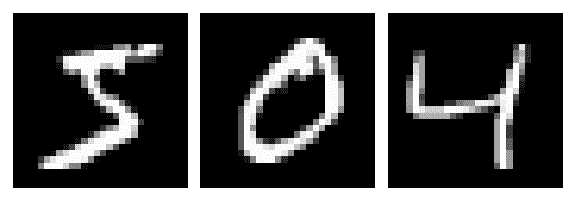Nielsen 提供了 MNIST 版本，为PKL.GZ（gzip 压缩的 Pickle）文件，可以轻松地将其加载到 Python 中。 出于本书 OpenCV 示例的目的，我们（作者）采用了 Nielsen 的 MNIST 的PKL.GZ版本，为我们的目的对其进行了重组，并将其放置在本书的chapter10/digits_data/mnist.pkl.gz的 GitHub 存储库中。

# 实现训练 ANN 的模块

1. 首先，我们将从 Python 标准库中导入gzippickle模块。 和往常一样，我们还将导入 OpenCV 和 NumPy：
import gzip
import pickle

import cv2
import numpy as np


((training_images, training_ids),
(test_images, test_ids))


1. 让我们编写以下帮助函数来解压缩并加载mnist.pkl.gz的内容：
def load_data():
mnist = gzip.open('./digits_data/mnist.pkl.gz', 'rb')
mnist.close()
return (training_data, test_data)


1. 我们必须重新格式化原始数据，以匹配 OpenCV 期望的格式。 具体来说，当我们提供用于训练 ANN 的样本输出时，它必须是具有 10 个元素（用于 10 类数字）的向量，而不是单个数字 ID。 为方便起见，我们还将应用 Python 内置的zip函数以一种可以对匹配的输入和输出向量对（如元组）进行迭代的方式来重组数据。 让我们编写以下辅助函数来重新格式化数据：
def wrap_data():
training_inputs = tr_d
training_results = [vectorized_result(y) for y in tr_d]
training_data = zip(training_inputs, training_results)
test_data = zip(te_d, te_d)
return (training_data, test_data)

1. 请注意，前面的代码调用load_data和另一个帮助函数vectorized_result。 后者将 ID 转换为分类向量，如下所示：
def vectorized_result(j):
e = np.zeros((10,), np.float32)
e[j] = 1.0
return e


1. 到目前为止，我们已经编写了用于加载和重新格式化 MNIST 数据的函数。 现在，让我们编写一个函数来创建未经训练的 ANN：
def create_ann(hidden_nodes=60):
ann = cv2.ml.ANN_MLP_create()
ann.setLayerSizes(np.array([784, hidden_nodes, 10]))
ann.setActivationFunction(cv2.ml.ANN_MLP_SIGMOID_SYM, 0.6, 1.0)
ann.setTrainMethod(cv2.ml.ANN_MLP_BACKPROP, 0.1, 0.1)
ann.setTermCriteria(
(cv2.TERM_CRITERIA_MAX_ITER | cv2.TERM_CRITERIA_EPS,
100, 1.0))
return ann


1. 现在，我们需要一个训练函数，允许调用者指定 MNIST 训练样本的数量和周期的数量。 我们以前的 ANN 样本应该熟悉很多训练函数，因此让我们看一下整个实现，然后再讨论一些细节：
def train(ann, samples=50000, epochs=10):

tr, test = wrap_data()

# Convert iterator to list so that we can iterate multiple
# times in multiple epochs.
tr = list(tr)

for epoch in range(epochs):
print("Completed %d/%d epochs" % (epoch, epochs))
counter = 0
for img in tr:
if (counter > samples):
break
if (counter % 1000 == 0):
print("Epoch %d: Trained on %d/%d samples" % \
(epoch, counter, samples))
counter += 1
sample, response = img
data = cv2.ml.TrainData_create(
np.array([sample], dtype=np.float32),
cv2.ml.ROW_SAMPLE,
np.array([response], dtype=np.float32))
if ann.isTrained():
ann.train(data, cv2.ml.ANN_MLP_UPDATE_WEIGHTS | cv2.ml.ANN_MLP_NO_INPUT_SCALE | cv2.ml.ANN_MLP_NO_OUTPUT_SCALE)
else:
ann.train(data, cv2.ml.ANN_MLP_NO_INPUT_SCALE | cv2.ml.ANN_MLP_NO_OUTPUT_SCALE)
print("Completed all epochs!")

return ann, test


1. 当然，经过训练的 ANN 的目的是进行预测，因此我们将提供以下predict函数，以便包装 ANN 自己的predict方法：
def predict(ann, sample):
if sample.shape != (784,):
if sample.shape != (28, 28):
sample = cv2.resize(sample, (28, 28),
interpolation=cv2.INTER_LINEAR)
sample = sample.reshape(784,)
return ann.predict(np.array([sample], dtype=np.float32))


def test(ann, test_data):
num_tests = 0
num_correct = 0
for img in test_data:
num_tests += 1
sample, correct_digit_class = img
digit_class = predict(ann, sample)
if digit_class == correct_digit_class:
num_correct += 1
print('Accuracy: %.2f%%' % (100.0 * num_correct / num_tests))


# 实现最小的测试模块

from digits_ann import create_ann, train, test

ann, test_data = train(create_ann())
test(ann, test_data)


Completed 0/10 epochs
Epoch 0: Trained on 0/50000 samples
Epoch 0: Trained on 1000/50000 samples
... [more reports on progress of training] ...
Completed all epochs!
Accuracy: 95.39%


# 实现主要模块

1. 首先，我们将导入 OpenCV，NumPy 和我们的digits_ann模块：
import cv2
import numpy as np

import digits_ann

1. 现在，让我们编写一些辅助函数来分析和调整数字和其他轮廓的边界矩形。 如前几章所述，重叠检测是一个常见问题。 以下称为inside的函数将帮助我们确定一个边界矩形是否完全包含在另一个边界矩形内：
def inside(r1, r2):
x1, y1, w1, h1 = r1
x2, y2, w2, h2 = r2
return (x1 > x2) and (y1 > y2) and (x1+w1 < x2+w2) and \
(y1+h1 < y2+h2)


1. 让我们看一下wrap_digit的实现。 首先，我们修改矩形的较小尺寸（宽度或高度），使其等于较大尺寸，然后修改矩形的xy位置，以使中心保持不变：
def wrap_digit(rect, img_w, img_h):

x, y, w, h = rect

x_center = x + w//2
y_center = y + h//2
if (h > w):
w = h
x = x_center - (w//2)
else:
h = w
y = y_center - (h//2)

1. 接下来，我们在所有侧面添加 5 像素填充：
    padding = 5


1. 为了避免超出范围的问题，我们对矩形进行裁剪，使其完全位于图像内。 在这些边缘情况下，这可能会给我们留下非正方形的矩形，但这是可以接受的折衷方案。 我们宁愿使用感兴趣的非正方形区域，而不是仅仅因为它位于图像的边缘而完全抛弃检测到的数字。 这是用于边界检查和裁剪矩形的代码：
    if x < 0:
x = 0
elif x > img_w:
x = img_w

if y < 0:
y = 0
elif y > img_h:
y = img_h

if x+w > img_w:
w = img_w - x

if y+h > img_h:
h = img_h - y

1. 最后，我们返回修改后的矩形的坐标：
    return x, y, w, h


1. 现在，让我们进入程序的主要部分。 在这里，我们首先创建一个 ANN 并在 MNIST 数据上对其进行训练：
ann, test_data = digits_ann.train(
digits_ann.create_ann(60), 50000, 10)


1. 现在，让我们在一张白纸上加载一个包含许多手写数字的测试图像：
img_path = "./digit_img/digits_0.jpg"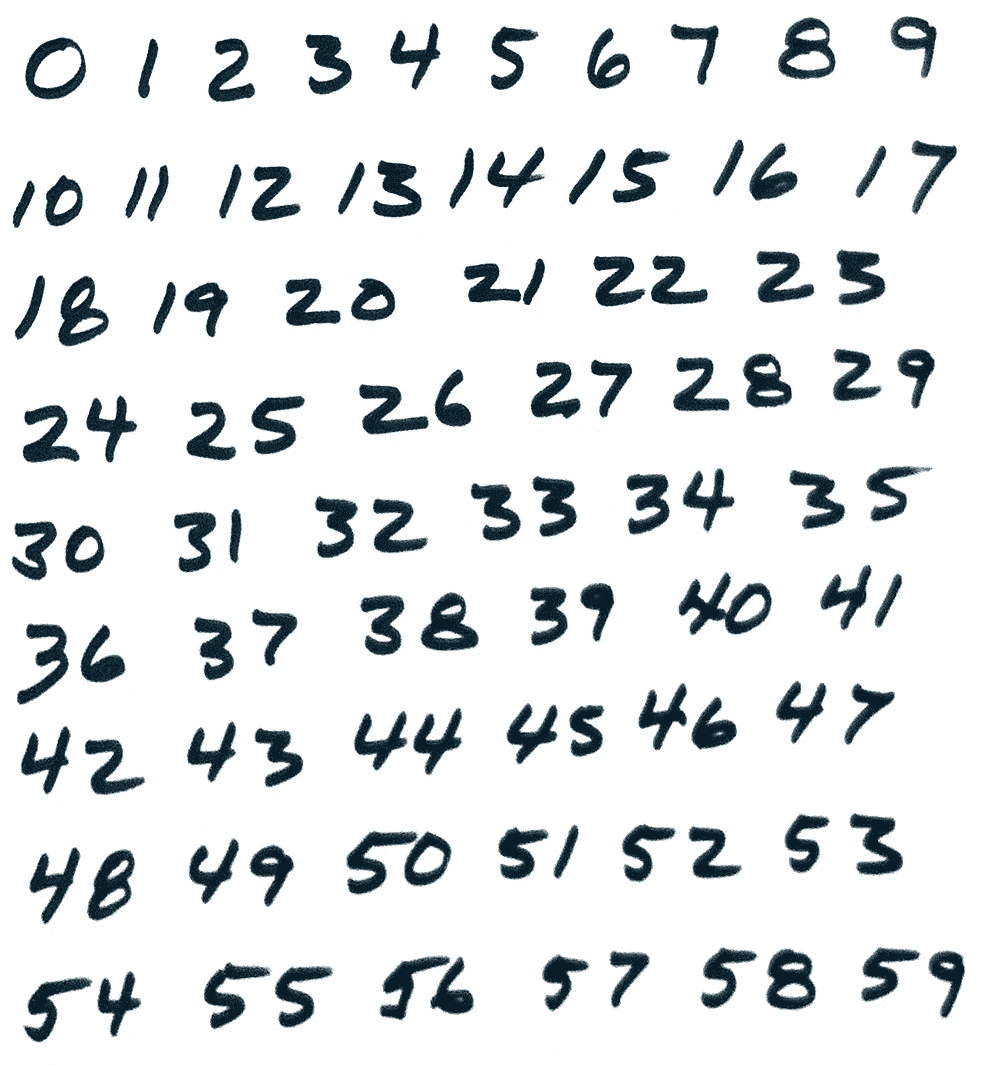1. 让我们将图像转换为灰度并使其模糊，以消除噪点并使墨水的暗度更加均匀：
gray = cv2.cvtColor(img, cv2.COLOR_BGR2GRAY)
cv2.GaussianBlur(gray, (7, 7), 0, gray)

1. 现在我们有了一个平滑的灰度图像，我们可以应用一个阈值和一些形态学操作，以确保数字与背景脱颖而出，并且轮廓相对没有不规则性，这可能会超出预测。 以下是相关代码：
ret, thresh = cv2.threshold(gray, 127, 255, cv2.THRESH_BINARY_INV)
erode_kernel = np.ones((2, 2), np.uint8)
thresh = cv2.erode(thresh, erode_kernel, thresh, iterations=2)


1. 进行形态学操作后，我们需要分别检测图片中的每个数字。 为此，首先，我们需要找到轮廓：
contours, hier = cv2.findContours(thresh, cv2.RETR_TREE,
cv2.CHAIN_APPROX_SIMPLE)

1. 然后，我们遍历轮廓并找到其边界矩形。 我们丢弃任何我们认为太大或太小而无法数字化的矩形。 我们还将丢弃完全包含在其他矩形中的所有矩形。 其余的矩形将追加到一个良好的矩形列表中（我们相信），这些矩形包含单个数字。 让我们看下面的代码片段：
rectangles = []

img_h, img_w = img.shape[:2]
img_area = img_w * img_h
for c in contours:

a = cv2.contourArea(c)
if a >= 0.98 * img_area or a <= 0.0001 * img_area:
continue

r = cv2.boundingRect(c)
is_inside = False
for q in rectangles:
if inside(r, q):
is_inside = True
break
if not is_inside:
rectangles.append(r)

1. 现在我们有了一个好的矩形列表，可以遍历它们，使用wrap_digit函数对它们进行清理，并对其中的图像数据进行分类：
for r in rectangles:
x, y, w, h = wrap_digit(r, img_w, img_h)
roi = thresh[y:y+h, x:x+w]
digit_class = int(digits_ann.predict(ann, roi))

1. 此外，在对每个数字进行分类之后，我们绘制了经过清理的边界矩形和分类结果：
    cv2.rectangle(img, (x,y), (x+w, y+h), (0, 255, 0), 2)
cv2.putText(img, "%d" % digit_class, (x, y-5),
cv2.FONT_HERSHEY_SIMPLEX, 1, (255, 0, 0), 2)

1. 处理完所有兴趣区域后，我们将保存阈值图像和带有完整标注的图像，并显示它们，直到用户按下任何键以结束程序为止：
cv2.imwrite("detected_and_classified_digits_thresh.png", thresh)
cv2.imwrite("detected_and_classified_digits.png", img)
cv2.imshow("thresh", thresh)
cv2.imshow("detected and classified digits", img)
cv2.waitKey()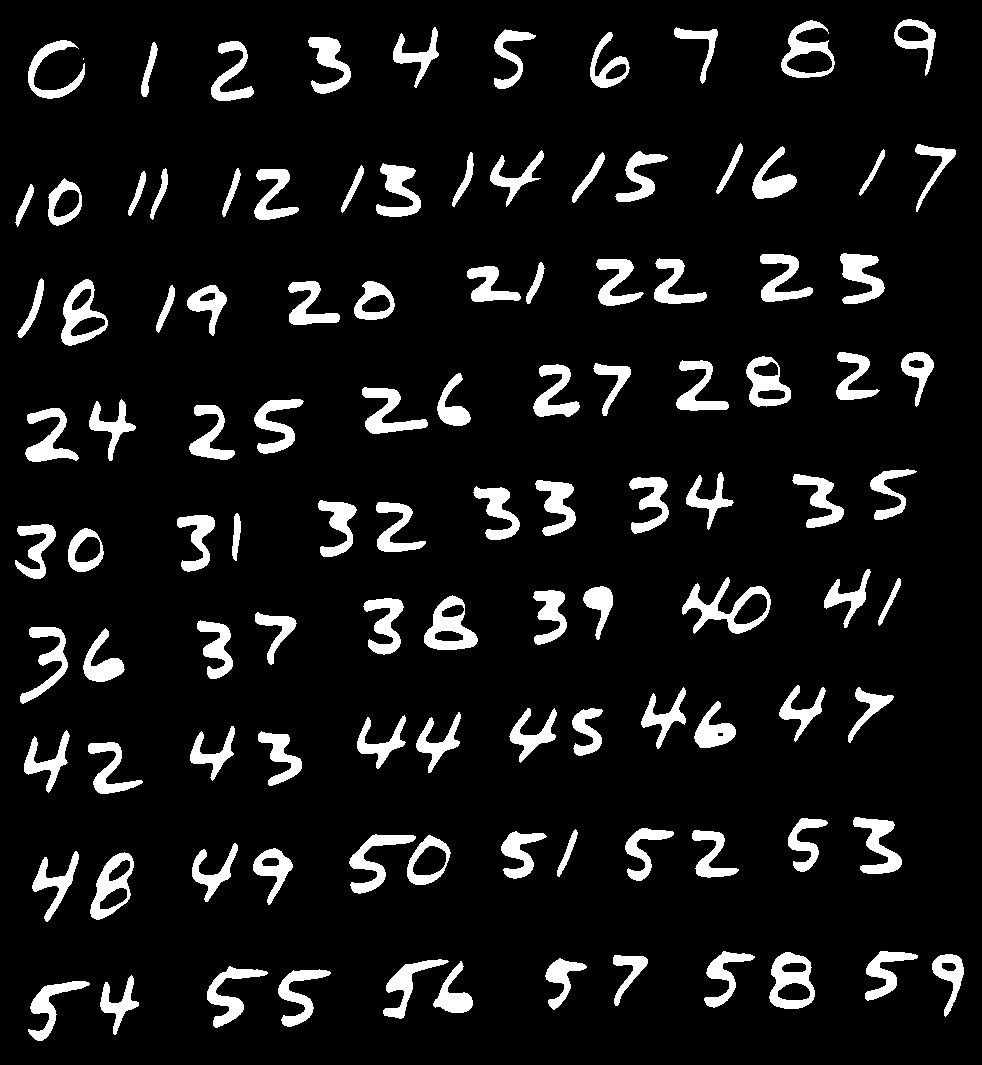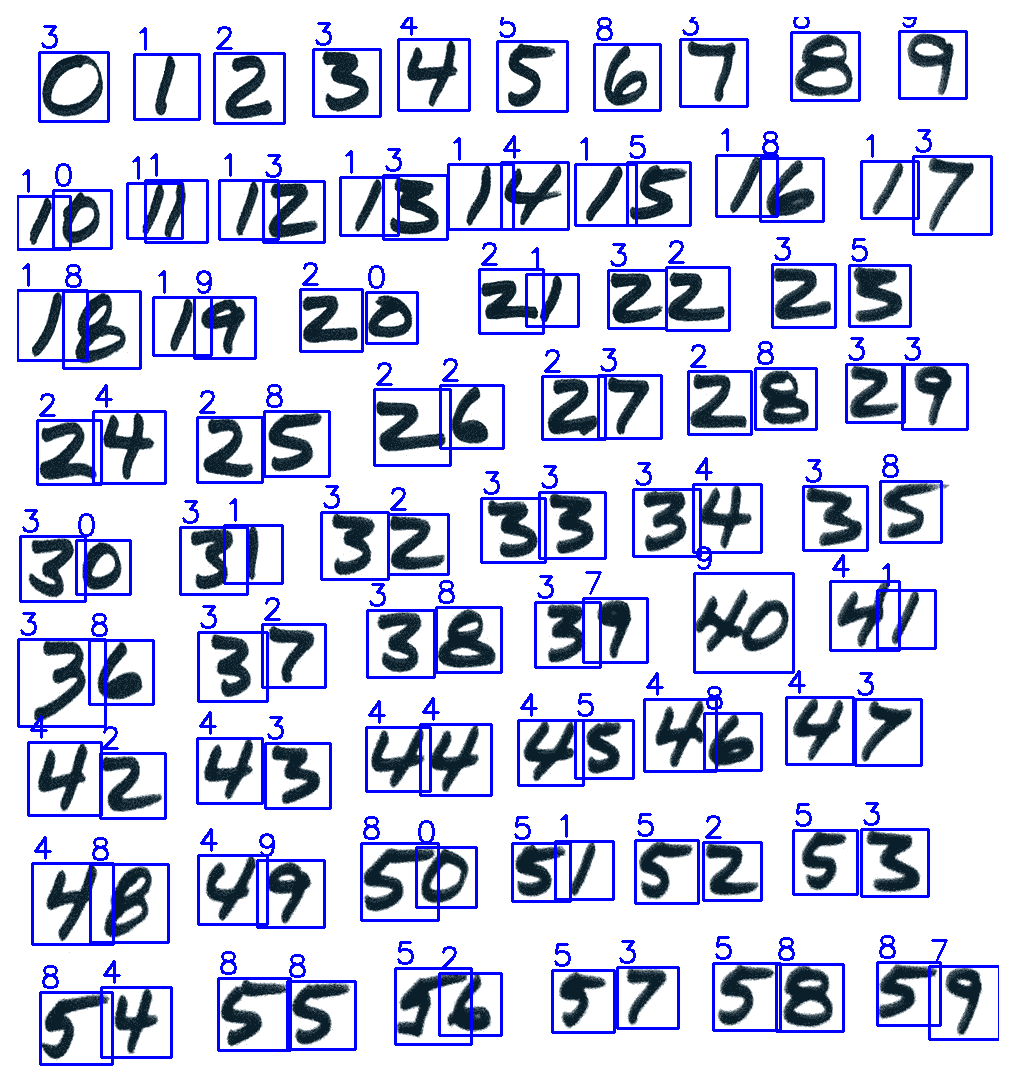# 试图改善人工神经网络的训练

• 您可以尝试训练数据集的大小，隐藏节点的数量和周期的数量，直到找到最高的准确率。
• 您可以修改digits_ann.create_ann函数，使其支持多个隐藏层。
• 您也可以尝试其他激活函数。 我们使用了cv2.ml.ANN_MLP_SIGMOID_SYM，但这不是唯一的选择。 其他包括cv2.ml.ANN_MLP_IDENTITYcv2.ml.ANN_MLP_GAUSSIANcv2.ml.ANN_MLP_RELUcv2.ml.ANN_MLP_LEAKYRELU
• 同样，您可以尝试不同的训练方法。 我们使用了cv2.ml.ANN_MLP_BACKPROP。 其他选项包括cv2.ml.ANN_MLP_RPROPcv2.ml.ANN_MLP_ANNEAL

MNIST 数据库是在美国编译的，数字 7 与手写字符 7 一样是手写的。但是，在欧洲，数字 7 通常是用数字的对角线部分中间的一条小水平线手写的。 引入此笔划是为了帮助区分手写数字 7 和手写数字 1。

ann = cv2.ml.ANN_MLP_create()
data = cv2.ml.TrainData_create(
training_samples, layout, training_responses)
ann.train(data)
ann.save('my_ann.xml')


ann = cv2.ml.ANN_MLP_create()


# 在 OpenCV 中使用其他框架的 DNN

OpenCV 可以加载和使用在以下任何框架中经过训练的 DNN：

caffe_model = cv2.dnn.readNetFromCaffe(
'my_model_description.protext', 'my_model.caffemodel')

'my_model.pb', 'my_model_description.pbtxt')

# Some Torch models use the .t7 extension and others use
# the .net extension.

'my_model_description.cfg', 'my_model.weights')

'my_model_description.xml', 'my_model.bin')


# 使用第三方 DNN 检测和分类对象

• MobileNetSSD_deploy.caffemodel：这是模型。
• MobileNetSSD_deploy.prototxt：这是描述模型参数的文本文件。

1. 与往常一样，我们首先导入 OpenCV 和 NumPy：
import cv2
import numpy as np

1. 我们以上一节中介绍的相同方式继续使用 OpenCV 加载 Caffe 模型：
model = cv2.dnn.readNetFromCaffe(
'objects_data/MobileNetSSD_deploy.prototxt',
'objects_data/MobileNetSSD_deploy.caffemodel')

1. 我们需要定义一些特定于该模型的预处理参数。 它期望输入图像为 300 像素高。 此外，它期望图像中的像素值在 -1.0 到 1.0 的范围内。 这意味着相对于从 0 到 255 的通常标度，有必要减去 127.5，然后除以 127.5。 我们将参数定义如下：
blob_height = 300
color_scale = 1.0/127.5
average_color = (127.5, 127.5, 127.5)

1. 我们还定义了一个置信度阈值，表示为了将检测作为真实对象而需要的最低置信度得分：
confidence_threshold = 0.5

1. 该模型支持 20 类对象，其 ID 为 1 到 20（而不是 0 到 19）。 这些类的标签可以定义如下：
labels = ['airplane', 'bicycle', 'bird', 'boat', 'bottle', 'bus',
'car', 'cat', 'chair', 'cow', 'dining table', 'dog',
'horse', 'motorbike', 'person', 'potted plant', 'sheep',
'sofa', 'train', 'TV or monitor']


1. 对于每一帧，我们首先计算纵横比。 请记住，此 DNN 期望输入基于 300 像素高的图像； 但是，宽度可以变化以匹配原始的宽高比。 以下代码段显示了如何捕获帧并计算适当的输入大小：
cap = cv2.VideoCapture(0)

while success:

h, w = frame.shape[:2]
aspect_ratio = w/h

# Detect objects in the frame.

blob_width = int(blob_height * aspect_ratio)
blob_size = (blob_width, blob_height)

1. 此时，我们可以简单地使用cv2.dnn.blobFromImage函数及其几个可选参数来执行必要的预处理，包括调整帧的大小并将其像素数据转换为 -1.0 到 1.0 的比例：
    blob = cv2.dnn.blobFromImage(
frame, scalefactor=color_scale, size=blob_size,
mean=average_color)

1. 我们将生成的 Blob 馈送到 DNN 并获取模型的输出：
    model.setInput(blob)
results = model.forward()


1. 对于此对象检测 DNN（以及使用 SSD 框架训练的其他 DNN），结果包括检测到的对象的子数组，每个对象都有自己的置信度得分，矩形坐标和类 ID。 以下代码显示了如何访问它们，以及如何使用 ID 在我们先前定义的列表中查找标签：
    # Iterate over the detected objects.
for object in results[0, 0]:
confidence = object
if confidence > confidence_threshold:

# Get the object's coordinates.
x0, y0, x1, y1 = (object[3:7] * [w, h, w, h]).astype(int)

# Get the classification result.
id = int(object)
label = labels[id - 1]

1. 遍历检测到的对象时，我们绘制检测矩形，分类标签和置信度得分：
            # Draw a blue rectangle around the object.
cv2.rectangle(frame, (x0, y0), (x1, y1),
(255, 0, 0), 2)

# Draw the classification result and confidence.
text = '%s (%.1f%%)' % (label, confidence * 100.0)
cv2.putText(frame, text, (x0, y0 - 20),
cv2.FONT_HERSHEY_SIMPLEX, 1, (255, 0, 0), 2)

1. 我们对框架所做的最后一件事就是展示它。 然后，如果用户按下Esc键，则退出； 否则，我们将捕获另一帧并继续循环的下一个迭代：
    cv2.imshow('Objects', frame)

k = cv2.waitKey(1)
if k == 27: # Escape
break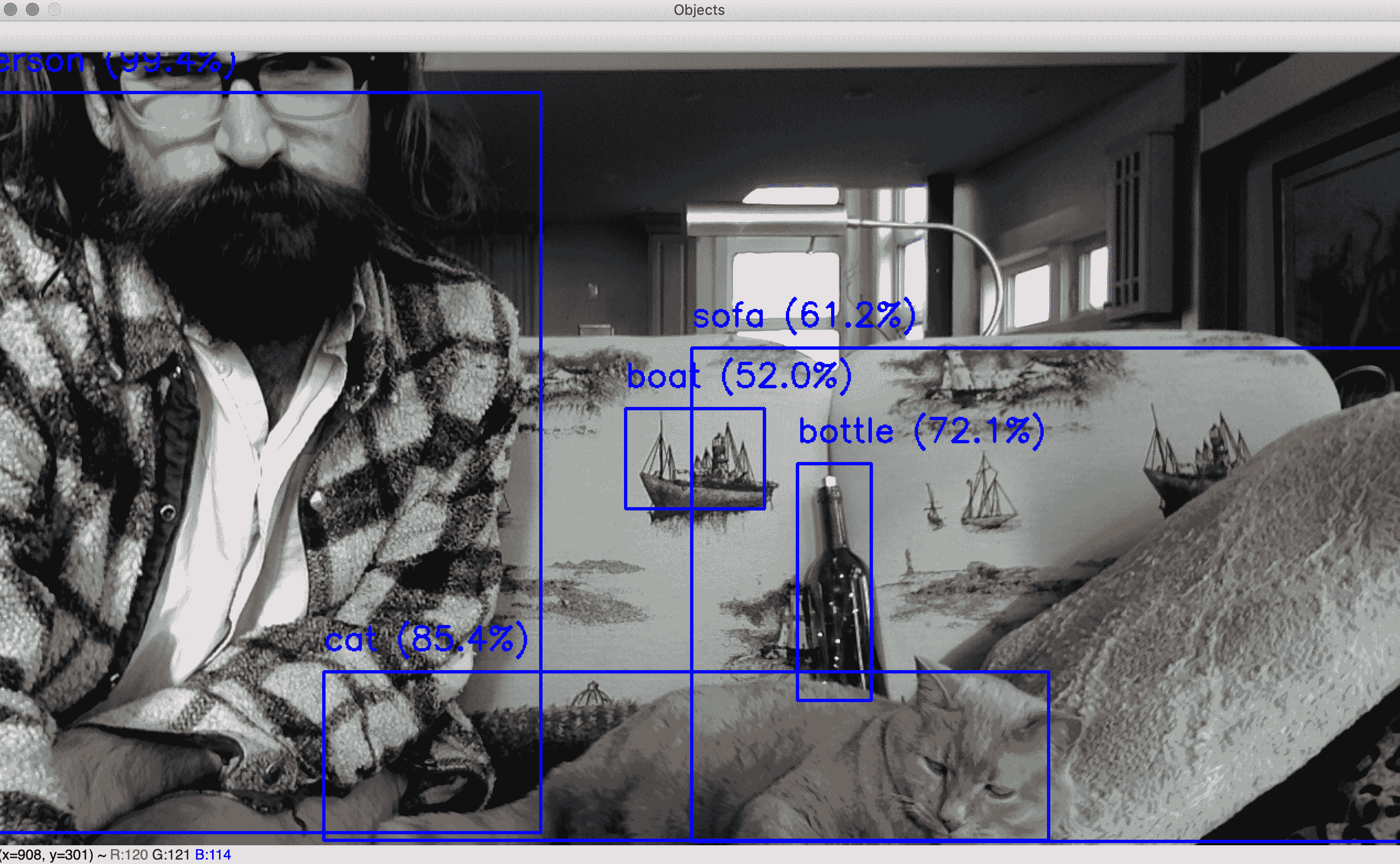DNN 已正确检测并分类了人类（置信度为 99.4%），（85.4%），装饰性瓶子（72.1%），并进行了分类， 沙发的一部分（61.2%），以及的纺织品图片（52.0%）。 显然，该 DNN 能够很好地对航海环境中的客厅进行分类！

# 使用第三方 DNN 检测和分类人脸

• detection/res10_300x300_ssd_iter_140000.caffemodel：这是用于人脸检测的 DNN。 OpenCV 团队已在这个页面提供了此文件。 这个 Caffe 模型是使用 SSD 框架训练的。 因此，它的拓扑类似于上一节示例中使用的 MobileNet-SSD 模型。
• detection/deploy.prototxt：这是文本文件，描述了用于人脸检测的先前 DNN 的参数。 OpenCV 团队在这个页面提供此文件。

chapter10/faces_data/age_gender_classification文件夹包含以下文件，这些文件均由 Gil Levi 和 Tal Hassner 在其 GitHub 存储库及其项目页面上提供，他们在年龄和性别分类方面的工作：

• age_net.caffemodel：这是用于年龄分类的 DNN。
• age_net_deploy.protext：这是文本文件，描述了用于年龄分类的先前 DNN 的参数。
• gender_net.caffemodel：这是用于性别分类的 DNN。
• gender_net_deploy.protext：这是文本文件，描述了用于年龄分类的先前 DNN 的参数。
• average_face.npyaverage_face.png：这些文件表示分类器训练数据集中的平均面孔。 来自 Levi 和 Hassner 的原始文件称为mean.binaryproto，但我们已将其转换为 NumPy 可读格式和标准图像格式，这对于我们的使用更加方便。

1. 为了开始示例程序，我们加载人脸检测 DNN，定义其参数，并定义置信度阈值。 我们以与上一节样本中的对象检测 DNN 大致相同的方式执行此操作：
import cv2
import numpy as np

'faces_data/detection/deploy.prototxt',
'faces_data/detection/res10_300x300_ssd_iter_140000.caffemodel')
face_blob_height = 300
face_average_color = (104, 177, 123)
face_confidence_threshold = 0.995


1. 现在，让我们加载年龄分类器并定义其分类标签：
age_model = cv2.dnn.readNetFromCaffe(
'faces_data/age_gender_classification/age_net_deploy.prototxt',
'faces_data/age_gender_classification/age_net.caffemodel')
age_labels = ['0-2', '4-6', '8-12', '15-20',
'25-32', '38-43', '48-53', '60+']


1. 现在，让我们加载性别分类器并定义其标签：
gender_model = cv2.dnn.readNetFromCaffe(
'faces_data/age_gender_classification/gender_net_deploy.prototxt',
'faces_data/age_gender_classification/gender_net.caffemodel')
gender_labels = ['male', 'female']

1. 年龄和性别分类器使用相同的 Blob 大小和相同的平均值。 他们使用的不是平均颜色，而是平均颜色的人脸图像，我们将从NPY文件中加载该图像（作为浮点格式的 NumPy 数组）。 稍后，我们将在执行分类之前从实际的面部图像中减去该平均面部图像。 以下是斑点大小和平均图像的定义：
age_gender_blob_size = (256, 256)
'faces_data/age_gender_classification/average_face.npy')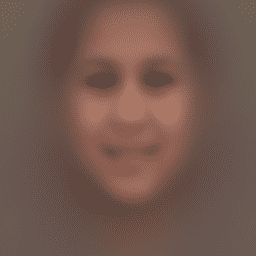1. 设置好模型及其参数后，让我们继续从相机捕获和处理帧。 对于每一帧，我们首先创建一个与帧相同的宽高比的 blob，然后将此 blob 馈送到人脸检测 DNN：
cap = cv2.VideoCapture(0)

while success:

h, w = frame.shape[:2]
aspect_ratio = w/h

# Detect faces in the frame.

face_blob_width = int(face_blob_height * aspect_ratio)
face_blob_size = (face_blob_width, face_blob_height)

face_blob = cv2.dnn.blobFromImage(
frame, size=face_blob_size, mean=face_average_color)

face_model.setInput(face_blob)
face_results = face_model.forward()

1. 就像我们在上一部分示例中使用的对象检测器一样，人脸检测器提供置信度得分和矩形坐标作为结果的一部分。 对于每个检测到的面部，我们需要检查置信度得分是否可以接受地高，如果是，则将获得面部矩形的坐标：
    # Iterate over the detected faces.
for face in face_results[0, 0]:
face_confidence = face
if face_confidence > face_confidence_threshold:

# Get the face coordinates.
x0, y0, x1, y1 = (face[3:7] * [w, h, w, h]).astype(int)

1. 此人脸检测 DNN 生成的矩形长于宽度。 但是，DNN 的年龄和性别分类要求使用方形面孔。 让我们加宽检测到的脸部矩形以使其成为正方形：
            # Classify the age and gender of the face based on a
# square region of interest that includes the neck.

y1_roi = y0 + int(1.2*(y1-y0))
x_margin = ((y1_roi-y0) - (x1-x0)) // 2
x0_roi = x0 - x_margin
x1_roi = x1 + x_margin
if x0_roi < 0 or x1_roi > w or y0 < 0 or y1_roi > h:
# The region of interest is partly outside the
# frame. Skip this face.
continue


1. 此时，我们可以选择正方形兴趣区域ROI），其中包含将用于年龄和性别分类的图像数据。 我们将 ROI 缩放到分类器的斑点大小，将其转换为浮点格式，然后减去平均脸部。 根据生成的缩放后的标准化脸部，创建斑点：
            age_gender_roi = frame[y0:y1_roi, x0_roi:x1_roi]
scaled_age_gender_roi = cv2.resize(
age_gender_roi, age_gender_blob_size,
interpolation=cv2.INTER_LINEAR).astype(np.float32)
scaled_age_gender_roi[:] -= age_gender_average_image
age_gender_blob = cv2.dnn.blobFromImage(
scaled_age_gender_roi, size=age_gender_blob_size)

1. 我们将斑点输入年龄分类器，选择具有最高置信度得分的类 ID，然后记下该 ID 的标签和置信度得分：
            age_model.setInput(age_gender_blob)
age_results = age_model.forward()
age_id = np.argmax(age_results)
age_label = age_labels[age_id]
age_confidence = age_results[0, age_id]

1. 同样，我们将性别分类：
            gender_model.setInput(age_gender_blob)
gender_results = gender_model.forward()
gender_id = np.argmax(gender_results)
gender_label = gender_labels[gender_id]
gender_confidence = gender_results[0, gender_id]

1. 我们绘制检测到的脸部矩形，扩展的方形 ROI 和分类结果的可视化图像：
            # Draw a blue rectangle around the face.
cv2.rectangle(frame, (x0, y0), (x1, y1),
(255, 0, 0), 2)

# Draw a yellow square around the region of interest
# for age and gender classification.
cv2.rectangle(frame, (x0_roi, y0), (x1_roi, y1_roi),
(0, 255, 255), 2)

# Draw the age and gender classification results.
text = '%s years (%.1f%%), %s (%.1f%%)' % (
age_label, age_confidence * 100.0,
gender_label, gender_confidence * 100.0)
cv2.putText(frame, text, (x0_roi, y0 - 20),
cv2.FONT_HERSHEY_SIMPLEX, 1, (0, 255, 255), 2)

1. 最后，我们显示带标注的帧，并继续捕获更多帧，直到用户按下Esc键：
cv2.imshow('Faces, age, and gender', frame)

k = cv2.waitKey(1)
if k == 27: # Escape
break